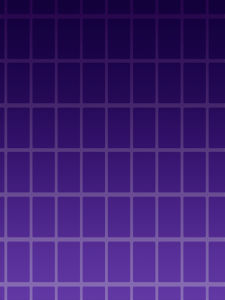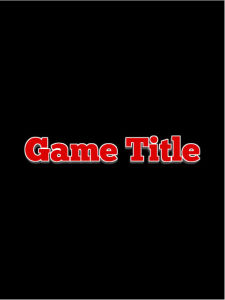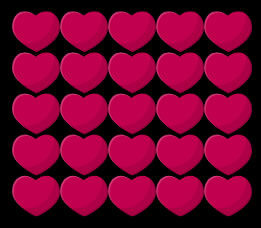# 5 Time Saving Phaser Classes

### Deja Vu All Over Again!

If you ever get a Deja Vu feeling when you are coding, it is probably time to put that code in some form of reusability! I found myself writing a lot of the same things over and over, so I built these classes for my personal library. Here are a few of my most used classes in Phaser.

## The Background

What it is: This class takes an image key and creates an image that fills the screen. It does not maintain proportions, so it may not work well with every image

How To Use

`var bg=new Background("imageKey");`

The Code

```class Background extends Phaser.Group {
constructor(key) {
super(game);
this.image.width = game.width;
this.image.height = game.height;
}
}```## The Logo

What it is: The logo component loads an image and gives it a center anchor and scales it proportionality to a percentage of the screen’s width.

I use this a lot when building title screens

How To Use

```//make a logo 80% of the game
var logo=new Logo("gameTitle",.8);```The Code

```class Logo extends Phaser.Group {
constructor(key, w = -1) {
super(game);
var image = game.add.image(0, 0, key);
image.anchor.set(0.5, 0.5);
if (w != -1) {
image.width = game.width * w;
image.scale.y = image.scale.x;
}
this.x = game.width / 2;
this.y = game.height / 2;
}
}```

## The Thumb

What it is: A Thumb component that loads a sprite and center anchor it. I use it a bit with the grid component below.

How To Use

`var thumb=new Thumb('heart');`If you need to add a listener you can access the icon inside the thumb with

```thumb.getIcon().eventEnabled=true;

The Code

```class Thumb extends Phaser.Group {
constructor(key) {
super(game);
this.icon.anchor.set(0.5, 0.5);
}
getIcon() {
return this.icon;
}
}```

## The Item Line

What it is: The item line allows you to create a horizontal or vertical line of sprites

How To Use

```var line=new ItemLine();

Add some items to the line.

for (var i = 0; i < 10; i++) {
Align.scaleToGameW(heart,.05);
}```

Then line it up vertically

`line.lineUpV();`or horizontally

`line.lineUpH();`The Code

```class ItemLine extends Phaser.Group {
constructor() {
super(game);
}
}
lineUpV() {
var len = this.children.length;
var yy = 0;
for (var i = 0; i < len; i++) {
var c = this.getChildAt(i);
c.y = yy;
yy += c.height;
}
}
lineUpH() {
var len = this.children.length;
var xx = 0;
for (var i = 0; i < len; i++) {
var c = this.getChildAt(i);
c.x = xx;
xx += c.width;
}
}

}```

## The Grid

What it is: The grid component allows you to quickly create a grid of items

How To Use

```var grid=new Grid();

Add some items to the grid

for (var i = 0; i < 25; i++) {
Align.scaleToGameW(heart,.1);
}

//Make a grid of 5 columns

grid.makeGrid(5);```The Code

```class Grid extends Phaser.Group {
constructor(xspace=1,yspace=1) {
super(game);
this.xspace=xspace;
this.yspace=yspace;
}
makeGrid(cols) {
var len = this.children.length;
var yy = 0;
var xx = 0;
for (var i = 0; i < len; i++) {
var c = this.getChildAt(i);
c.x = xx * c.width*this.xspace;
c.y = yy * c.height*this.yspace;
xx++;
if (xx == cols) {
xx = 0;
yy++;
}
}
}
}```

I hope you find these as helpful as I have

You can download all of these as part of the Ultimate Game Parts Template